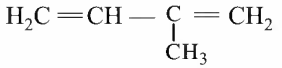Buna-N synthetic rubber is a copolymer of

# Buna-N synthetic rubber is a copolymer of

1. A

${\mathrm{H}}_{2}\mathrm{C}=\mathrm{CH}-\mathrm{CN}$ and ${\mathrm{H}}_{2}\mathrm{C}=\mathrm{CH}-\mathrm{CH}={\mathrm{CH}}_{2}$

2. B

${\mathrm{H}}_{2}\mathrm{C}=\mathrm{CH}-\mathrm{CN}$ and3. C

and ${\mathrm{H}}_{2}\mathrm{C}=\mathrm{CH}-\mathrm{CH}={\mathrm{CH}}_{2}$

4. D

${\mathrm{H}}_{2}\mathrm{C}=\mathrm{CH}-\mathrm{CH}={\mathrm{CH}}_{2}$ and ${\mathrm{H}}_{5}{\mathrm{C}}_{6}-\mathrm{CH}={\mathrm{CH}}_{2}$

Fill Out the Form for Expert Academic Guidance!l

+91

Live ClassesBooksTest SeriesSelf Learning

Verify OTP Code (required)

### Solution:

Buna−N is a synthetic rubber copolymer made up of 1,3−Butadiene (H2​C=CHCH=CH2​) and acrylonitrile (H2​C=CHCN).

The synthesis is as follows: Buna−N is a synthetic rubber copolymer made up of 1,3−Butadiene (H2​C=CHCH=CH2​) and acrylonitrile (H2​C=CHCN).

The synthesis is as follows:

${\mathrm{H}}_{2}\mathrm{C}=\mathrm{CH}-\mathrm{CN}$ and ${\mathrm{H}}_{2}\mathrm{C}=\mathrm{CH}-\mathrm{CH}={\mathrm{CH}}_{2}$+91

Live ClassesBooksTest SeriesSelf Learning

Verify OTP Code (required)